Computer modeling allows using computational methods, such as, finite element technique, finite difference method, finite volume method, estimate, behaves like an object - the building, gasser, road embankment, pipeline and other support. - In actual use. Simulation helps to ensure the efficiency and reliability of the design solutions with minimal time and material costs.

For computer simulation of artificial freezing of soils used specialized software - Frost 3D Universal, where via numerical methods made solution of the heat equation with boundary conditions:Where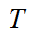- temperature;- time;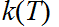- temperature dependence of thermal conductivity;- volumetric heat capacity of water;- filtration rate;- heat sources and sinks;- heat transfer coefficient;- the ambient temperature;- source of thermal radiation temperature;- the emissivity of the surface of the soil;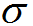- Stefan - Boltzmann constant;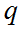- heat flow, for example with evaporator cooling unit.

Effective heat and thermal conductivity of soil can be defined as a function of temperature or calculated for the corresponding equations.The dependence of the heat capacity of the temperature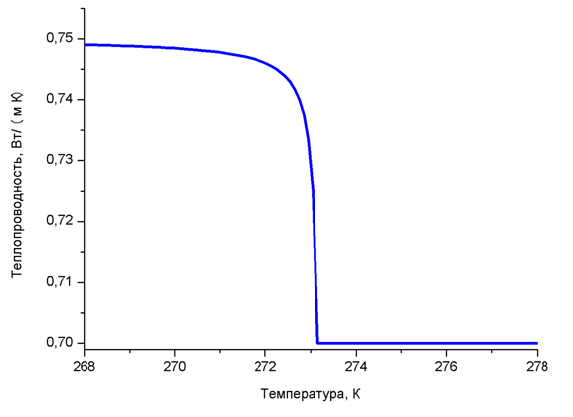The dependence of the thermal conductivity of the temperature

The most widely used for the calculation of the effective heat capacity and thermal conductivity obtained equation of the form:Where- the thermal conductivity of unfrozen and frozen ground, respectively,;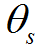- volumetric soil moisture content;- iciness dependence on temperature, which can be approximated by the following empirical relation: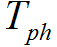- temperature phase transition ice-water;- empirical coefficient, which determines the slope of the temperature changes in the ice content in the vicinity of the phase transition.

The heat equation is solved with the boundary conditions for the three-dimensional domain, which is analyzed in the design object. This area, usually, It includes geological and lithological soil layers, projected bases and foundations, insulating layers, Device for cooling elements of the soil and other.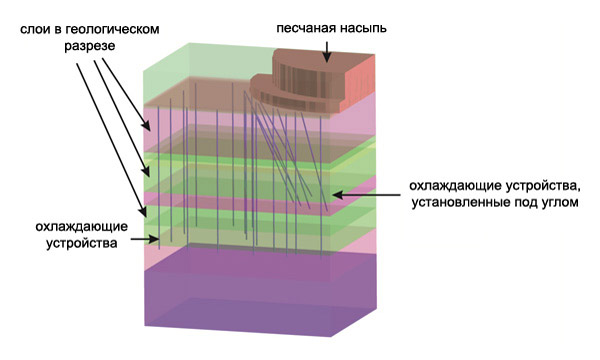Field site modeling for artificial soil freezing

For the upper limit of the simulation area, usually, taking the earth's surface, and the lower limit of a sufficiently large depth, in order to minimize its impact on thermal processes in the area of ​​interest to the designer.

The upper boundary of the simulation region defined conditions of heat exchange with the environment, are determined by the air temperature and heat transfer coefficient. In the presence of heat sources, eg, flare gas flaring, further defined by the temperature of the radiation source and the degree of black soil surface. The influence of snow cover on the heat transfer surface of the soil is carried out by setting the change in time of snow cover thickness and its thermal conductivity.

At the lower boundary of the simulation region is set according to ground temperature thermometric data. On the side surface of the modeling area, usually, given zero heat flow. In this simulation side of the boundary should be located far enough away from the area of ​​interest of the designer, so that they do not affect the calculation of thermal processes.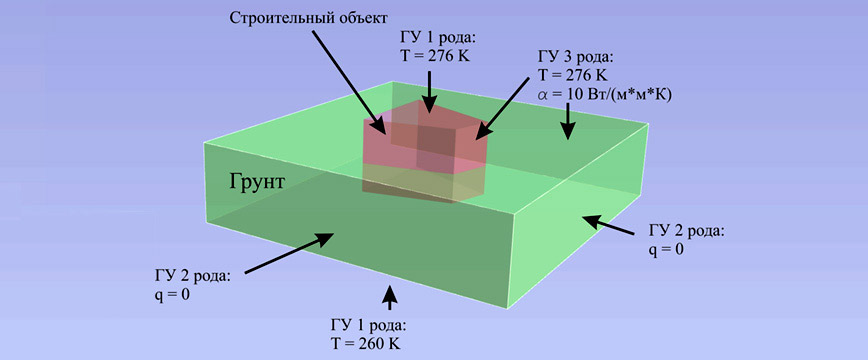Setting the boundary conditions on the modeling area in predicting the thermal regime of soils

It should be noted, that the area of ​​modeling consists of a non-homogeneous soil layers, with different physical properties - the specific heat, thermal conductivity, density and moisture content. In this connection, for computer simulation of artificial freezing of soils is necessary to construct a three-dimensional modeling, which takes into account the real distribution of soil layers with different thermal properties. This distribution of ground layers constructed on the basis of, obtained by geotechnical boreholes. When the complex structure of the soil to restore the modeling must be used geostatistical methods.

Restoration of geological and lithological structure of soils on the basis of geotechnical boreholes

As for the solution of the heat equation in a three-dimensional setting is necessary to use numerical methods, domain simulation sampled computational grid. For modeling of thermal processes in soils for large spatial scales optimum use hexahedral computational grid. As a result, the sample user-defined thermal properties of the soil and the boundary conditions are transferred to the corresponding elements and facets of the elements of the computational grid.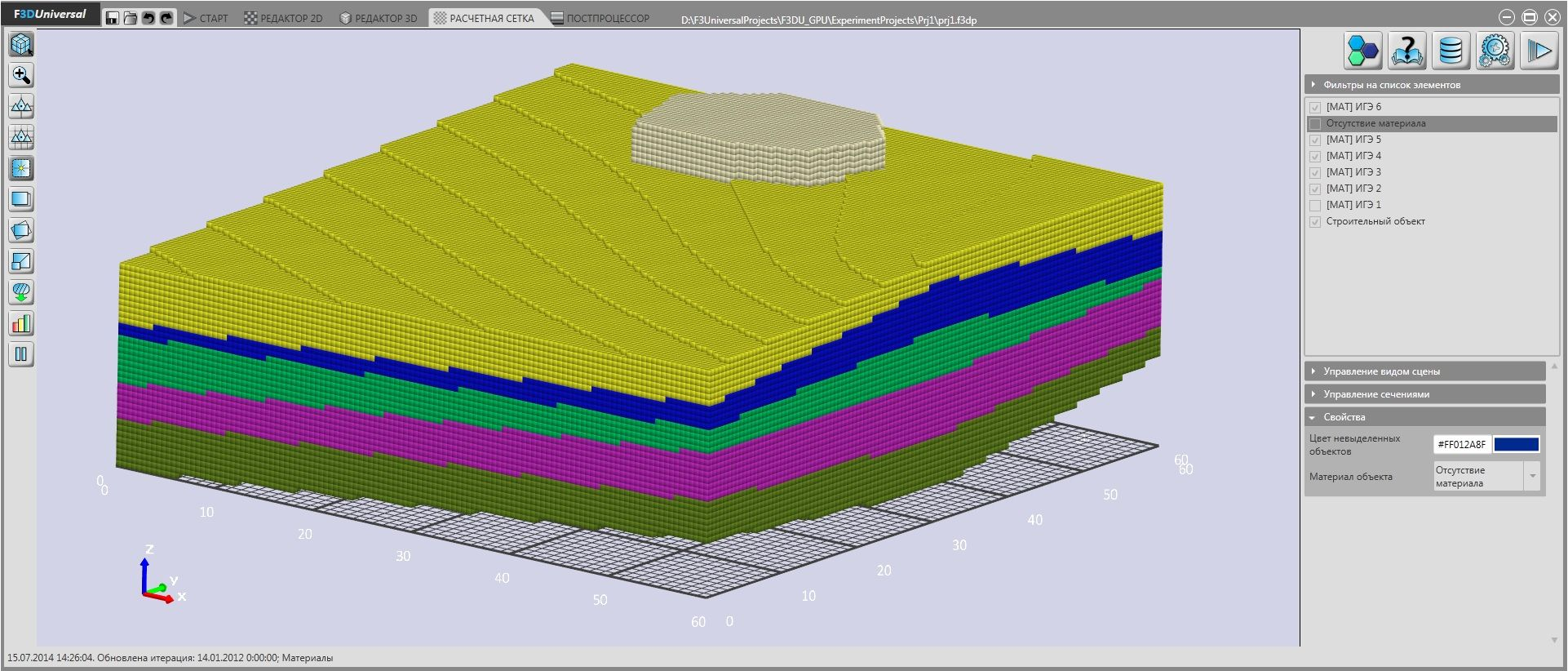Sampling field hexahedral mesh modeling

As a result, the numerical solution of the heat equation is obtained a three-dimensional temperature distribution in the modeled area for various times. The resulting thermal field is used to analyze the thermal conditions of soil and change their mechanical properties. Based on the analysis of the decision to ensure that the bearing capacity of soil.